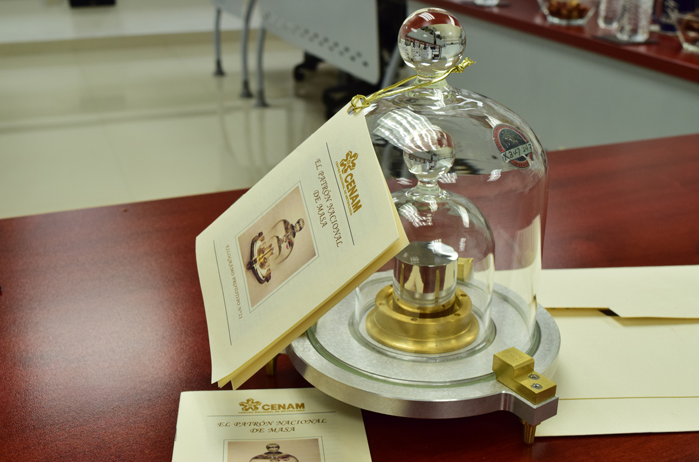Chemistry is based on observation and experimentation, trying to obtain a series of results that in many cases can be expressed numerically, allowing their comparison with those obtained in other experiences.

In all observation and experimentation we look at those properties that are susceptible to comparison and that, therefore, we can measure. These properties are called magnitudes.

There is a small group of magnitudes, called fundamentals, from which all the others can be obtained. These magnitudes are: length, mass, time, current intensity, temperature, amount of substance and light intensity.

The international system of units assigns a unit to each magnitude. The meter is the unit of length. If when measuring a distance, 20m is obtained, it means that the length is 20 times the unit of the international system.

The definitions of the fundamental units in the international system, in accordance with the corresponding resolutions of the General Conference on Weights and Measures (CGMP), are the following:

1.- Unit of length. The meter is the distance that light travels in a vacuum in 1/299,792,486 seconds. Approximately 39.37 inches. A meter is divided into 100 centimeters and 1000 millimeters.
2.- Unit of mass. The kilogram is the mass of a cylinder of platinum and iridium kept at Sevres, near Paris, France. The pound has a mass of 0.4536 kilograms. The kilogram is divided into 1000 grams.

3.- Unit of time. The second is the duration of 9,192,631,770 times the period of the radiation corresponding to the transition between the two hyperfine levels of the ground state of the cesium 133 atom.

4.- Unit of electric current intensity. The ampere is the intensity of a constant current that, maintained between two parallel, rectilinear conductors of infinite length, of negligible circular section and placed at a distance of one meter from each other, in a vacuum, produces between these conductors a force of 2 10 -7 newtons per meter of length.

5.- Thermodynamic temperature unit. The kelvin is the fraction 1/273.16 of the thermodynamic temperature of the triple point of water.

6.- Unit of quantity of substance. The mole is the amount of substance in a system that contains as many elementary entities as there are atoms in 12 grams of carbon 12.

7.- Luminous intensity unit. The candela is the luminous intensity, in the perpendicular direction, of a surface of 1/600,000 square meters of a black body at the freezing temperature of platinum under the pressure of 101,325 newtons per square meter.

Some magnitudes such as electrical intensity, luminous intensity, are little used in chemistry.